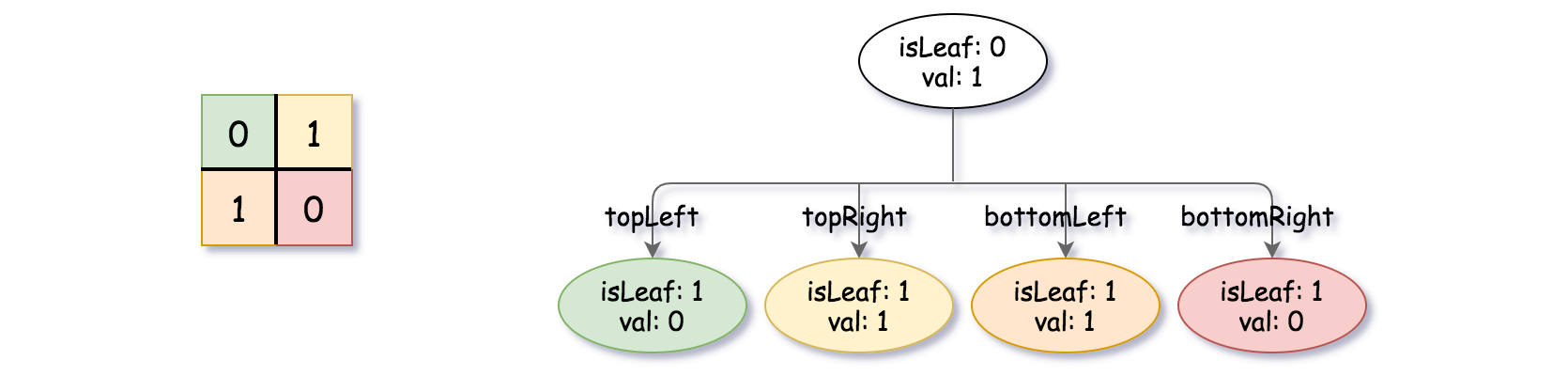# Leetcode 427 - Construct Quad Tree

Note:

• Divide and conquer, or DFS
• Use len to represent the current grid, initialized as n.
• If it’s all 0s or 1s in the grid, return a leaf.
• If not, divide the length by 2!! (Len would never be something like 6).
• Need params for starting point topLeft. Use i, j as start point.
• Start from there and expand it until we’ve found inharmonious vals or reached the bondaries.
• If it is the former case, divide the length by 2 and continue dfs on the 4 children grids.
• If it is the latter case, return a leaf node.

Question:

Given a n * n matrix grid of 0‘s and 1‘s only. We want to represent the grid with a Quad-Tree.

Return the root of the Quad-Tree representing the grid.

Notice that you can assign the value of a node to True or False when isLeaf is False, and both are accepted in the answer.

A Quad-Tree is a tree data structure in which each internal node has exactly four children. Besides, each node has two attributes:

• val: True if the node represents a grid of 1’s or False if the node represents a grid of 0’s.
• isLeaf: True if the node is leaf node on the tree or False if the node has the four children.

We can construct a Quad-Tree from a two-dimensional area using the following steps:

1. If the current grid has the same value (i.e all 1‘s or all 0‘s) set isLeaf True and set val to the value of the grid and set the four children to Null and stop.
2. If the current grid has different values, set isLeaf to False and set val to any value and divide the current grid into four sub-grids as shown in the photo.
3. Recurse for each of the children with the proper sub-grid.

Quad-Tree format:

The output represents the serialized format of a Quad-Tree using level order traversal, where null signifies a path terminator where no node exists below.

It is very similar to the serialization of the binary tree. The only difference is that the node is represented as a list [isLeaf, val].

If the value of isLeaf or val is True we represent it as 1 in the list [isLeaf, val] and if the value of isLeaf or val is False we represent it as 0.

Example:Code: